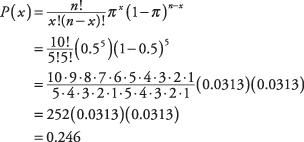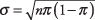## The Binomial

A discrete variable that can result in only one of two outcomes is called binomial. For example, a coin flip is a binomial variable, but drawing a card from a standard deck of 52 is not. Whether a drug is successful or unsuccessful in producing results is a binomial variable, as is whether a machine produces perfect or imperfect widgets.

#### Binomial experiments

Binomial experiments require the following elements:
• The experiment consists of a number of identical events ( n).

• Each event has only one of two mutually exclusive outcomes. (These outcomes are called successes and failures.)

• The probability of a success outcome is equal to some percentage, which is identified as a proportion, π.

• This proportion, π, remains constant throughout all events and is defined as the ratio of number of successes to number of trials.

• The events are independent.

• Given all the above, the binomial formula can be applied ( x = number of favorable outcomes; n = number of events):##### Example 1

A coin is flipped ten times. What is the probability of getting exactly five heads? Using the binomial formula, where n (the number of events) is given as 10; x (the number of favorable outcomes) is given as 5; and the probability of landing a head in one flip is 0.5:So, the probability of getting exactly five heads in ten flips is 0.246, or approximately 25 percent.

#### Binomial table

Because probabilities of binomial variables are so common in statistics, tables are used to alleviate having to continually use the formula. Refer to Table 1 in "Statistics Tables," and you will find that given n = 10, x = 5, and π = 0.5, the probability is 0.2461.

#### Mean and standard deviation

The mean of the binomial probability distribution is determined by the following formula:

μ = nπ

where π is the proportion of favorable outcomes and n is the number of events.

The standard deviation of the binomial probability distribution is determined by this formula:What is the mean and standard deviation for a binomial probability distribution for ten coin flips of a fair coin?

Because the proportion of favorable outcomes of a fair coin falling heads (or tails) is π = 0.5, simply substitute into the formulas:The probability distribution for the number of favorable outcomes is shown in Figure 1.

Note that this distribution appears to display symmetry. Only a binomial distribution with π = 0.5 will be truly symmetric. All other binomial distribution will be skewed.

Figure 1.The binomial probability distribution of the number of heads resulting from ten coin tosses.